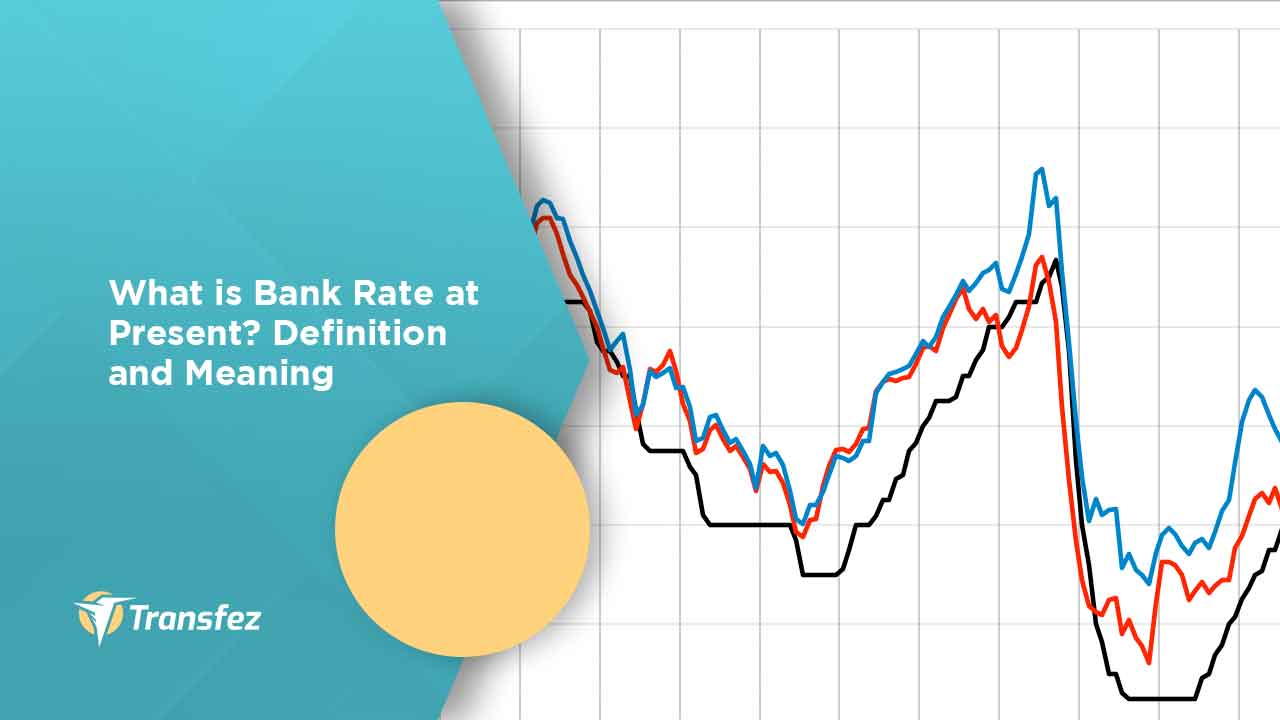Knowing everything about banks is often not easy and makes many people feel confused. One of them is when talking about what is a bank rate at present. Especially since this bank rate is not always the same value over time. So of course, it takes a sharp analysis to find out information about it.

However, for some bank customers, of course, knowing the size of the bank rate is important. Because this will be related to all financial activities carried out at the bank. Therefore, getting a little information about this will certainly be quite meaningful. More precisely here is information about what is bank rate at present and the amount of its current value.

List of ContentFirst of all, before knowing more about the details of what is bank rate at present, of course, it will be important to know first about the bank rate itself. Understanding bank interest rates is a fee charged as reciprocity between the bank and the customer is using financial products. The calculation of interest rates is usually expressed in the percentage amount. The amount of interest that must be paid either by the customer or the bank is based on bank rules and economic conditions.

Based on the loading method, bank interest rates are divided into four types. At least you should know this type of bank interest rate because the use of different methods will affect the amount of interest you have to pay. Interest rates will affect monthly installments that are principal and interest loans. The types of flowers based on the method of loading are:

### • Flat rate

A flat rate is the amount of interest charged each month that is fixed and calculated from the initial principal amount of the loan. Similarly, the principal installment of the loan is divided equally each month, so that the total installments each month are also the same until the credit is paid off.

### • Sliding rate

The amount of interest from the sliding rate each month is calculated from the rest of the loan so that the amount of interest paid by customers each month decreases along with the decrease in the principal of the loan. However, the principal payment of the loan every month is the same. Customer installments (principal plus interest) automatically decrease every month. This interest rate is also called effective interest. This type of credit is often found in long-term investment credits.

### • Annuity Rate

This interest rate is one of the categories of effective interest rates, where the amount of interest charged on each monthly credit installment is calculated mathematically so that the total installments each month are always the same value and the interest expense is calculated from the rest of the loan each month. This type of interest is found in the provision of homeownership loans.

### • Floating rate

While floating rate is the interest rate of credit that is associated with the prevailing interest in the market, the interest paid each month is very dependent on the market interest rate in that month. The amount of interest paid may be higher or lower or equal than the previous month. This type of interest is found in working capital loans and investment credits.

## About Bank Rate at Present

From the explanation of the bank rate above, there is also a so-called bank rate at present. Where this is the amount of bank rate that is being used by all banks in general at the same time. So that the value will be the same between the bank rate in one bank with another bank.

Information about bank rate at present is of course important for customers who want to get certain financial services from the bank. Especially if it is related to a particular loan or debt that will be taken out by the customer at that time. Knowing the size of the bank rate at present, of course, provides information about how much interest rates will be charged against loans taken by customers.Therefore, you should know the bank rate that is currently running is important. For information for the amount of bank rate applied by various banks is now at 4.25%. Which is the value of the bank rate at present refers to the amount of bank rate suggested by the central banks of various countries. This value is also based on information and calculations that have been done according to MSF or Marginal Standing Facility standards.

Thus, all banks operating under the central bank are obliged to set the value of the bank rate at present on all financial transaction instruments. Later the value can change at a later time depending on the results of the assessment conducted the next day.